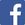## Calcrostic Puzzle

We call these problems calcrostic because they are showing calculations in a form similar to acrostic word puzzles. The color in the puzzle is used to indicate the computations in the rows, columns and diagonals. In a calcrostic, like:

``` ab × cd = ebaf
+ ×  ÷ ÷    −
egf ×  h =  hdc
= =  = =    =
ejc ×  k =  bfj
```

each letter represents a digit (one of 0,1,2,3,4,5,6,7,8,9). Equal letters represent equal digits, different letters represent different digits. The 9 resulting numbers ab, cd, ebaf, egf, h, hdc, ejc, k, bfj may have more than one digit (for example, ab is a 2-digit number) and are arranged in a 3 × 3 square as above. These numbers satisfy 3 horizontal, 3 vertical and 2 diagonal relations (like the diagonal relation ab × h = bfj where × stands for multiplication and ÷ for division. The problem is to find the value of each letter.

For example, the puzzle above has the solution

``` 54 × 27 = 1458
+ ×  ÷ ÷    −
108 ×  9 =  972
= =  = =    =
162 ×  3 =  486
```

Have fun!